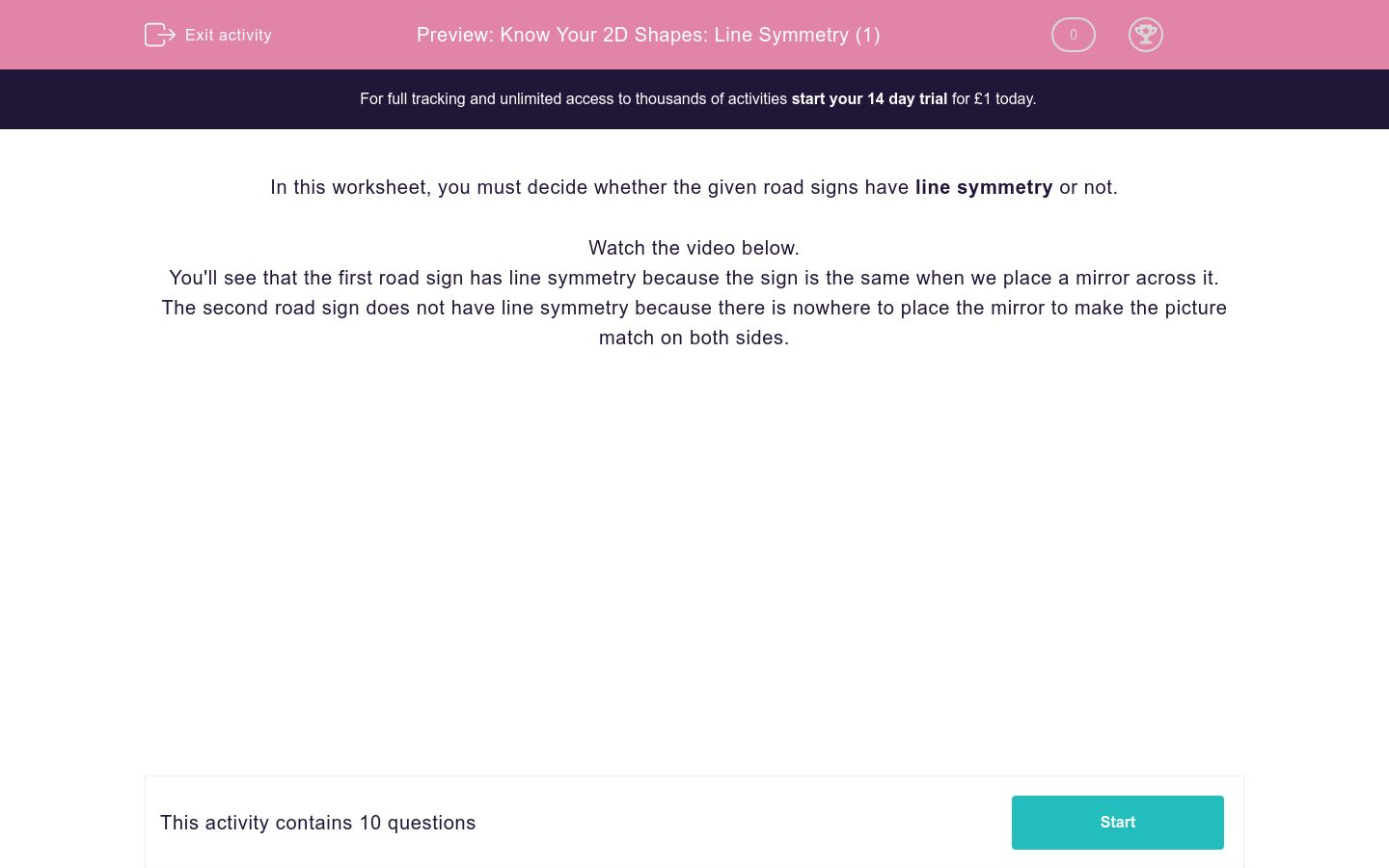# Know Your 2D Shapes: Line Symmetry (1)

In this worksheet, students study 2D diagrams for line symmetry.Key stage:  KS 2

Curriculum topic:   Maths and Numerical Reasoning

Curriculum subtopic:   Symmetry

Difficulty level:### QUESTION 1 of 10

What is symmetry?

Symmetry means that one shape becomes exactly like another when you move it in some way: turn, flip or slide.For two objects to be symmetrical, they must be the same size and shape,

What is Line Symmetry?

You may have heard this referred to as reflection symmetry?  This means that one half is an exact reflection of the other half.

The "Line of Symmetry" is the (dotted line) imaginary line where you could fold the image and have both halves match exactly.  Can you see how it works in the image below?In this worksheet, you must decide whether the given road signs have line symmetry or not.

---- OR ----